Published

# SMS based Home Automation system using 1SHEELD

Make a 5-channel SMS based Home Automation system using 1Sheeld.

BeginnerFull instructions provided31,110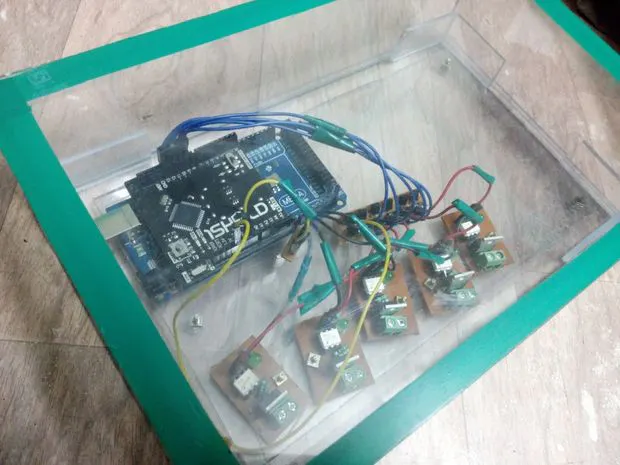## Things used in this project

### Hardware components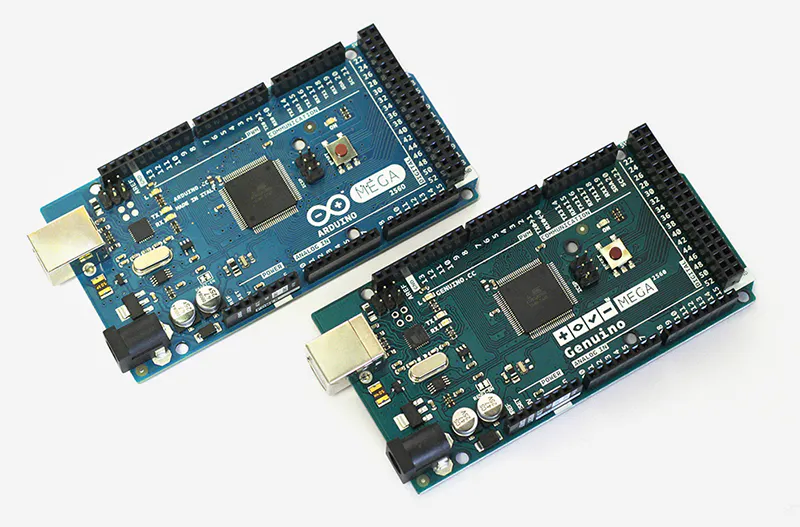Arduino Mega 2560 & Genuino Mega 2560
×1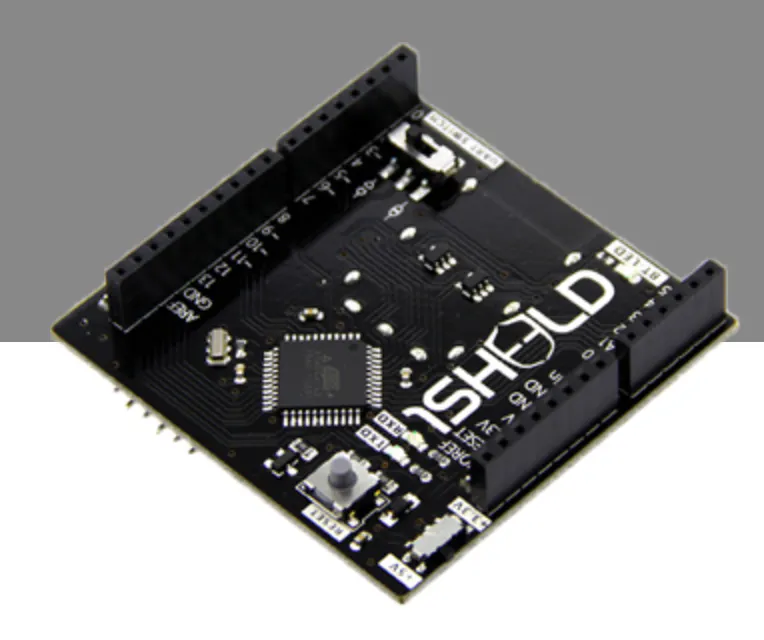1Sheeld
×1
 Relay (generic)
×5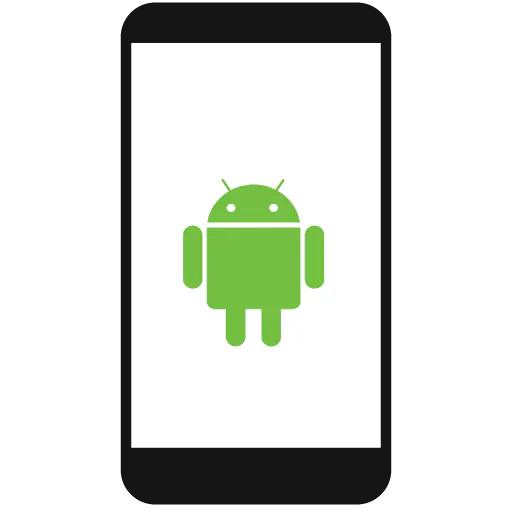Android device
×1

### Software apps and online servicesArduino IDE

## Schematics

### F4IRKCTI9IBNAU4.LARGE.jpg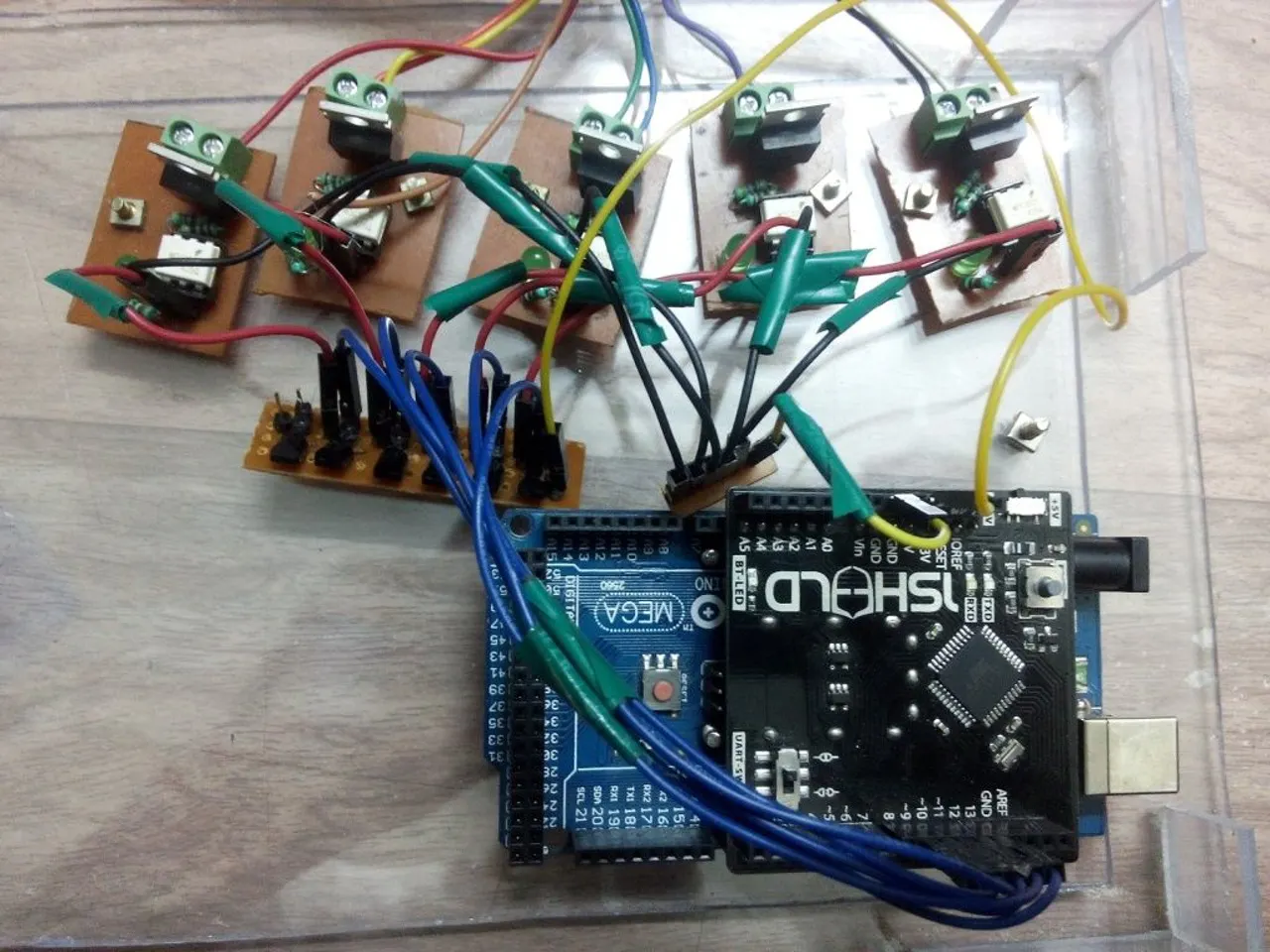## Code

### sms.txt

Plain text
```/*
A simple Example which will turn the pins on and
off while recieving specific messages from specific
phone.
5 pins which are used here pin: 13,12,11,10 and 9.

*/</p><p>    /* Include 1Sheeld Library */
#include <OneSheeld.h>
int ledPin13 =13 ;        /* led on pin 13 for debuging */
int ledPin12 =12;         /* led on pin 12 for debuging */
int ledPin11 =11;         /* led on pin 11 for debuging */
int ledPin10 =10;         /* led on pin 10 for debuging */
int ledPin9 =9;           /* led on pin 9 for debuging */
int ledpin =4;            /* led on pin 4 for debuging */</p><p>    void setup()
{
/* Start communication */
OneSheeld.begin();
/* This function invokeds receiveSms once any new messages are
}</p><p>    /* Nothing to loop on */
void loop()
{}</p><p>    /* Receiving Function for new SMS's */
void receiveSms(const char * number ,const char * text)
{
if(!strcmp(number,"+1234567890"))               /* phone number from which you will send the sms "PUT YOURS IN PLACE OF +1234567890" */
{
if(!strcmp(text,"on13"))                        /* Check if i need to open the light connected to (pin 13)*/
{
digitalWrite(ledPin13,HIGH);                  /* turn on the light (pin 13) */
}
if(!strcmp(text,"off13"))
{
digitalWrite(ledPin13,LOW);                   /* turn off the light(pin 13) */
}

if(!strcmp(text,"on12"))                        /* Check if i need to open the light (pin 12) */
{
digitalWrite(ledPin12,HIGH);                  /* turn on the light (pin 12) */
}
if(!strcmp(text,"off12"))

{
digitalWrite(ledPin12,LOW);                   /* turn off the light(pin 12) */
}

if(!strcmp(text,"on11"))                        /* Check if i need to open the light (pin 11) */
{
digitalWrite(ledPin11,HIGH);                  /* turn on the light (pin 11) */
}
if(!strcmp(text,"off11"))

{
digitalWrite(ledPin11,LOW);                   /* turn off the light(pin 11) */
}
if(!strcmp(text,"on10"))                        /* Check if i need to open the light (pin 10) */
{
digitalWrite(ledPin10,HIGH);                  /* turn on the light (pin 10) */
}
if(!strcmp(text,"off10"))

{
digitalWrite(ledPin10,LOW);                   /* turn off the light(pin 10) */
}
if(!strcmp(text,"on9"))                         /* Check if i need to open the light (pin 9) */
{
digitalWrite(ledPin9,HIGH);                   /* turn on the light (pin 9) */
}
if(!strcmp(text,"off9"))

{
digitalWrite(ledPin9,LOW);                    /* turn off the light(pin 9) */
}

if(!strcmp(text,"on all"))

{
digitalWrite(ledPin9,HIGH);          /* turn on the light(pin 9) */
digitalWrite(ledPin10,HIGH);         /* turn on the light(pin 9) */
digitalWrite(ledPin11,HIGH);         /* turn on the light(pin 9) */
digitalWrite(ledPin12,HIGH);         /* turn on the light(pin 9) */
digitalWrite(ledPin13,HIGH);         /* turn on the light(pin 9) */
}
if(!strcmp(text,"off all"))

{
digitalWrite(ledPin9,LOW);          /* turn off the light(pin 9) */
digitalWrite(ledPin10,LOW);         /* turn off the light(pin 9) */
digitalWrite(ledPin11,LOW);         /* turn off the light(pin 9) */
digitalWrite(ledPin12,LOW);         /* turn off the light(pin 9) */
digitalWrite(ledPin13,LOW);         /* turn off the light(pin 9) */
}
else
{
digitalWrite(ledpin,LOW);                    /* put any unused pin here (pin 4) */
}
}
}
```

### Untitled file

Arduino
```/*
A simple Example which will turn the pins on and
off while recieving specific messages from specific
phone.
5 pins which are used here pin: 13,12,11,10 and 9.

*/</p><p>    /* Include 1Sheeld Library */
#include <OneSheeld.h>
int ledPin13 =13 ;        /* led on pin 13 for debuging */
int ledPin12 =12;         /* led on pin 12 for debuging */
int ledPin11 =11;         /* led on pin 11 for debuging */
int ledPin10 =10;         /* led on pin 10 for debuging */
int ledPin9 =9;           /* led on pin 9 for debuging */
int ledpin =4;            /* led on pin 4 for debuging */</p><p>    void setup()
{
/* Start communication */
OneSheeld.begin();
/* This function invokeds receiveSms once any new messages are
}</p><p>    /* Nothing to loop on */
void loop()
{}</p><p>    /* Receiving Function for new SMS's */
void receiveSms(const char * number ,const char * text)
{
if(!strcmp(number,"+1234567890"))               /* phone number from which you will send the sms "PUT YOURS IN PLACE OF +1234567890" */
{
if(!strcmp(text,"on13"))                        /* Check if i need to open the light connected to (pin 13)*/
{
digitalWrite(ledPin13,HIGH);                  /* turn on the light (pin 13) */
}
if(!strcmp(text,"off13"))
{
digitalWrite(ledPin13,LOW);                   /* turn off the light(pin 13) */
}

if(!strcmp(text,"on12"))                        /* Check if i need to open the light (pin 12) */
{
digitalWrite(ledPin12,HIGH);                  /* turn on the light (pin 12) */
}
if(!strcmp(text,"off12"))

{
digitalWrite(ledPin12,LOW);                   /* turn off the light(pin 12) */
}

if(!strcmp(text,"on11"))                        /* Check if i need to open the light (pin 11) */
{
digitalWrite(ledPin11,HIGH);                  /* turn on the light (pin 11) */
}
if(!strcmp(text,"off11"))

{
digitalWrite(ledPin11,LOW);                   /* turn off the light(pin 11) */
}
if(!strcmp(text,"on10"))                        /* Check if i need to open the light (pin 10) */
{
digitalWrite(ledPin10,HIGH);                  /* turn on the light (pin 10) */
}
if(!strcmp(text,"off10"))

{
digitalWrite(ledPin10,LOW);                   /* turn off the light(pin 10) */
}
if(!strcmp(text,"on9"))                         /* Check if i need to open the light (pin 9) */
{
digitalWrite(ledPin9,HIGH);                   /* turn on the light (pin 9) */
}
if(!strcmp(text,"off9"))

{
digitalWrite(ledPin9,LOW);                    /* turn off the light(pin 9) */
}

if(!strcmp(text,"on all"))

{
digitalWrite(ledPin9,HIGH);          /* turn on the light(pin 9) */
digitalWrite(ledPin10,HIGH);         /* turn on the light(pin 9) */
digitalWrite(ledPin11,HIGH);         /* turn on the light(pin 9) */
digitalWrite(ledPin12,HIGH);         /* turn on the light(pin 9) */
digitalWrite(ledPin13,HIGH);         /* turn on the light(pin 9) */
}
if(!strcmp(text,"off all"))

{
digitalWrite(ledPin9,LOW);          /* turn off the light(pin 9) */
digitalWrite(ledPin10,LOW);         /* turn off the light(pin 9) */
digitalWrite(ledPin11,LOW);         /* turn off the light(pin 9) */
digitalWrite(ledPin12,LOW);         /* turn off the light(pin 9) */
digitalWrite(ledPin13,LOW);         /* turn off the light(pin 9) */
}
else
{
digitalWrite(ledpin,LOW);                    /* put any unused pin here (pin 4) */
}
}
}
```

### Github

https://github.com/Integreight/1Sheeld-Arduino-Library